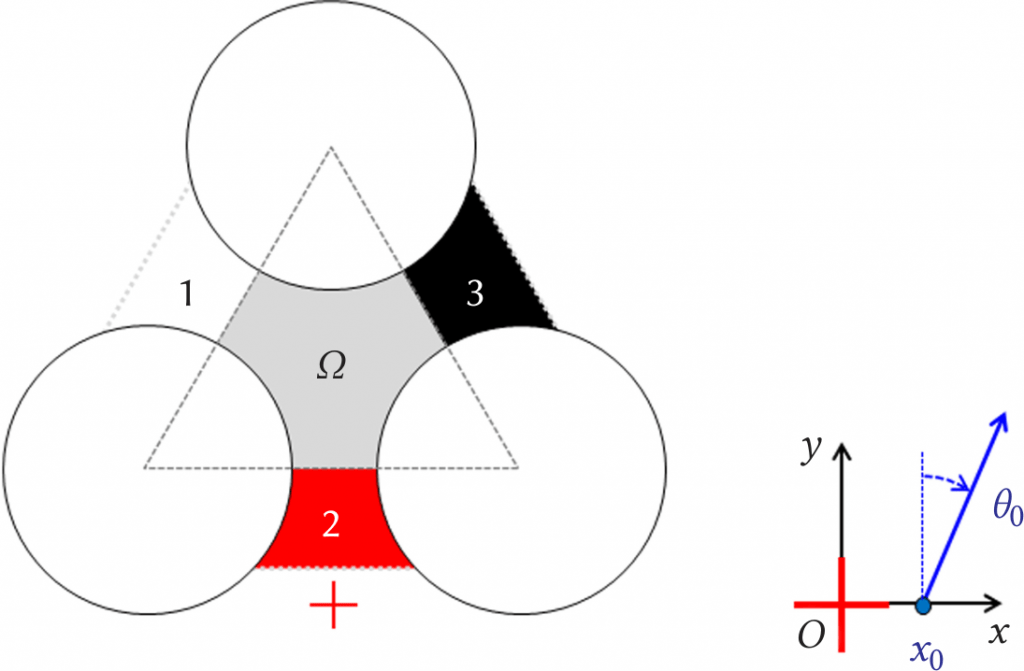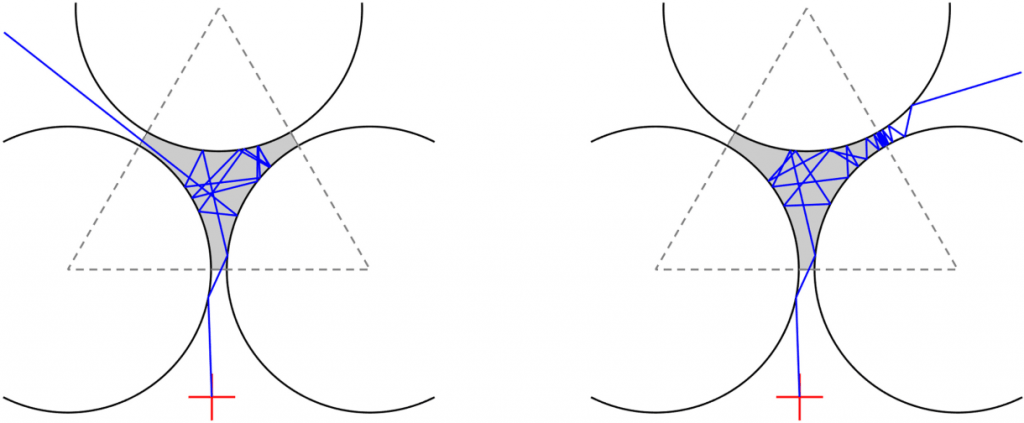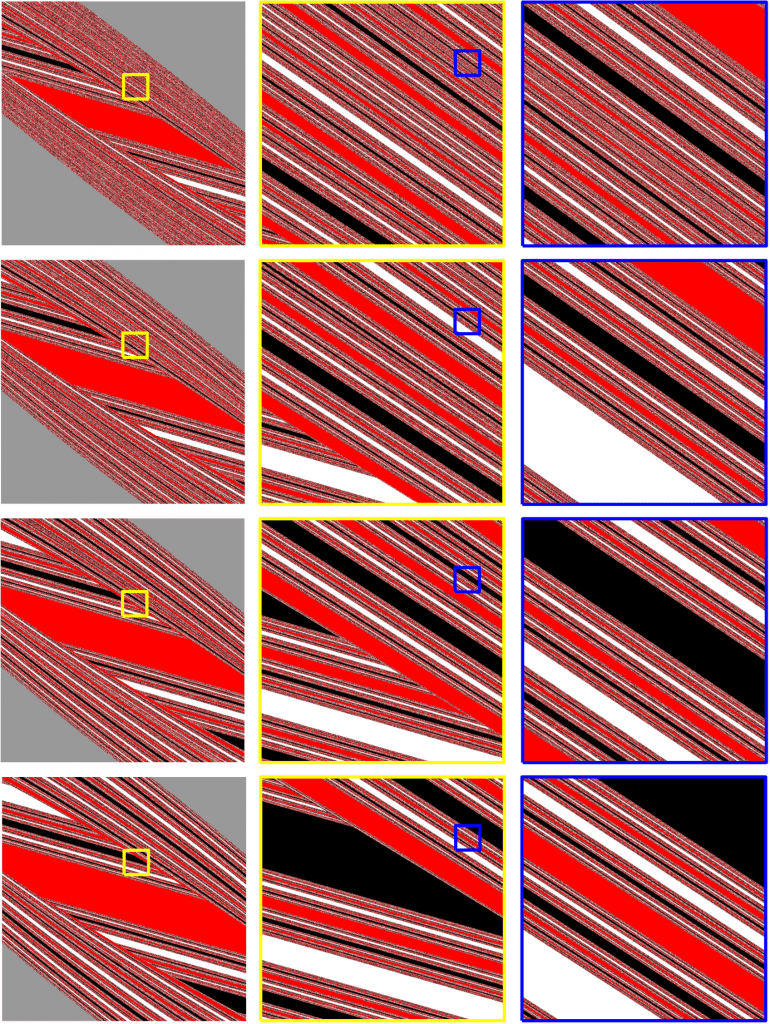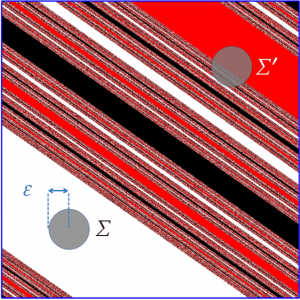# Chaotic scattering: uncertainty and fractals from reflections

James M Christian reflects on chaosFlickr user Windell Oskay (CC BY 2.0)

Any Chalkdust reader who has spent time playing billiards, snooker, or pool, will probably have some kind of feel for how the balls move around the table, how they bounce off each other, and how they bounce off the cushions. After a while, you just know instinctively how they should behave, at least in very simple situations. Reading this article is unlikely to improve your potting statistics; writing it has certainly not improved mine.

We can start by shrinking the cue ball to the size of a point, and imagining the object ball being squashed into a hard-edged circular disc that is so heavy that it does not recoil in a collision. The path of the incoming cue ball can be represented by a ray. Rays always follow straight lines between bounces, mimicking a ball rolling across a perfectly smooth horizontal table. Reflection of the incident ray by the disc is shown on the right. Draw a line from the disc’s centre through the point where the ray hits the circumference. That line is the normal, and the law of specular reflection tells us that angle out equals angle in, or in symbols, $\theta_\text{ref} = \theta_\text{inc}$.

To make things a bit more interesting, place identical discs at the corners of an equilateral triangle. That setup was first analysed in detail around three decades ago by physicist Pierre Gaspard and chemist Stuart Rice. Scientifically, their groundbreaking paper provided a paradigm for chaotic scattering in a plane but we can think of it as just an abstract game of pinball. The three-disc system turns out to be really useful in applied mathematics for understanding billiard-type problems, while in physics it crops up in fields from laser optics to the kinetic theory of gases.

## Formulating the problem

The three-disc arrangement is shown below. Each disc has a radius of 1 unit, the size of the gap between them is $\ell$, and the shaded area $\Omega$ is called the scattering region. Any incoming ray is defined by an incidence angle $\theta_0$ and a displacement $x_0$. We can select whatever values we like for $\theta_0$ and $x_0$, and will refer to the pair of numbers $(x_0,\theta_0)$ as the input.

An incident ray can generally end up doing only one of four things, which are the allowed outputs of the system. If the ray enters $\Omega$, it ricochets between the discs before eventually escaping through one of the three gaps. The fourth possible outcome is that the ray is reflected away early on and so never enters $\Omega$ at all. Sometimes, a ray may also remain trapped inside $\Omega$ forever! But that situation is so incredibly unlikely that we can discard it here. Our question to try and answer is: for a given input, what will the ray do?The three-disc system with the gaps labelled 1 to 3 and colour-coded, with the red cross showing the origin of $(x,y)$ coordinates. The incident ray has initial position $x_0$ and angle $\theta_0$ (positive angles are measured in the clockwise sense).

## The butterfly effect

The zig-zagging path of any ray (its trajectory) can be computed using straight lines and applying the law of specular reflection every time it hits a disc. Crucially, our scattering problem is deterministic because it is governed by mathematical rules in which there is absolutely no randomness whatsoever.

Given an input, classical physics demands that there must exist a unique output. Seems reasonable and obvious. What is not so reasonable and obvious is that a tiny change to the input can lead to a dramatic change in the output. This strange phenomenon—where a system can be susceptible to minuscule fluctuations—is known technically as sensitive dependence on initial conditions. The term ‘butterfly effect’ is perhaps more widely known, coined by mathematician and meteorologist Edward Lorenz in the early 1970s as a nod to the unpredictability he discovered in a toy model of the weather. It purports, only half-jokingly, that a butterfly flapping its wings in Brazil can set off tornadoes in Texas.

The butterfly effect appears in our scattering problem when we have, for example, two incoming rays starting from the same $x_0$ value but with slightly different $\theta_0$ values. The difference between their paths may become magnified through successive bounces, building up remarkably quickly until the two trajectories have diverged and no longer bear any resemblance to one another. Ultimately, we may even find that the two almost (but not quite!) identical inputs lead to completely different outputs.A demonstration of the butterfly effect in the three-disc system. On the right, $\theta_0$ has been increased by 0.001° compared to the left.

Scattering that exhibits the butterfly effect is said to be chaotic. In common parlance, ‘chaotic’ is often used to convey disorder or perhaps even (perceived) randomness. Here, we are deploying the word in a scientific sense. While it might sometimes look random, the three-disc system cannot do anything except behave deterministically. It also hides some very intricate and very ordered structure that can be seen if we look in the right place$\dots$

## Exit basins and their properties

Ideally, we would like to relate a whole range of inputs to their corresponding outputs in one go. A nice way to do that is to think of $x_0$ and $\theta_0$ as labelling the horizontal and vertical axes, respectively, in a plane (just like the $x$–$y$ axes in coordinate geometry). Let us impose a square grid on a section of that $(x_0,\theta_0)$ plane, where all the points represent different initial conditions. For each point in turn, the outcome of the computation is recorded. The collection of outputs is then overlaid on top of the grid, and colour-coded according to our three-disc system in figure 1 to create a kind of map.Figure 2: A demonstration of the butterfly effect in the three-disc system. On the right, $\theta_0$ has been increased by 0.001° compared to the left.

An example is shown below when the gap between the discs is relatively small. The map answers our earlier question in a very direct and effective way. It tells us exactly what a ray does as a function of starting point $(x_0,\theta_0)$, and even cuts out all the unnecessary information about individual trajectories. But a single map is just the tip of a giant iceberg, and many more questions now follow.Figure 3: Exit basins when the gap width gradually increases (top to bottom: $\ell = 0.07$, $0.09$, $0.11$, and $0.13$) and the plane of initial conditions $(x_0,\theta_0)$ in the left-hand column is $[-0.1,0.1] \times [-10°,10°]$.

We can see that the $(x_0,\theta_0)$ plane is divided into four coloured regions, known as exit basins, which identify a unique output for a given input—white for gap 1, red for gap 2, black for gap 3 (initial conditions giving rise to trajectories that never enter $\Omega$ are shown in grey). The boundaries of these basins form striated patterns that are intertwined in extremely complicated ways. An important feature of the map is its self-similarity, where zooming-in on any portion of a boundary region uncovers smaller-scale substructure which looks like the pattern as a whole. Self-similarity persists all the way down to arbitrarily-fine length scales, and that property is typically one of the signatures of a mathematical fractal.

It has been known since the mid 1990s that the basins can also possess the infinitely-mindbending Wada property which, in visual terms, means the boundary between two colours never quite forms. Amazingly, the remaining colours somehow always nestle in. Wada says that we can never jump from a black region to a red region without also jumping across a white region. Similar is true for other black-red-white permutations. More subtly, any jump over a boundary necessarily involves crossing all three colours an infinite number of times!

As an analogy to the Wada property, think about the points on a number line. Any number is either rational (expressible as a ratio of integers, like $1/2$ or $3/4$) or irrational ($\pi$ or $\sqrt{2}$ are the usual suspects). We cannot jump from $1/2$ to $3/4$ without necessarily jumping over all the numbers in between, both the (countably-infinite) rationals and the (uncountably-infinite) irrationals. The same idea would also hold if we were to jump between any two irrational numbers instead.

It turns out that the basins vary with the gap width $\ell$ (see below). For instance, the map takes up more of the $(x_0,\theta_0)$ plane as $\ell$ increases. That result makes physical sense: we would expect the scattering region to be accessible from a wider range of initial conditions as the gaps become bigger. We can also see, qualitatively, that the pattern complexity reduces as $\ell$ increases since the density of the striations (loosely speaking, the number of stripes per unit area of boundary) drops off as the gaps expand.

The striations in the maps look quite linear, especially when magnified. However, some curving can be seen if we look over a wider range of the $(x_0,\theta_0)$ plane than shown here, particularly when the gap size increases.

There is also a fundamental physical principle determining how the stripes must fit together (perhaps you spotted it earlier). Look on the right: if the black and white areas are swapped over, the map still looks the same! This is a consequence of the fact that there is no difference between gaps 1 and 3. Our three-disc system has a line of mirror symmetry along the $y$-axis, and so the trajectories from $(-x_0,-\theta_0)$ and $(x_0,\theta_0)$ are necessarily mirror images of each other.

## Uncertainty and unpredictability

So far, we have found that the butterfly effect can play a key role in scattering, and that basins become less finely-structured as the gaps increase in size. Far from being independent, these attributes are two sides of the same coin. One way to establish their connection is through the concept of uncertainty, thinking more carefully about what it means for a deterministic system to have an uncertain outcome. How can that apparent contradiction be allowed by physics?!

Let us start with an arbitrary initial condition, say $\boldsymbol{x}_0 \equiv (x_0,\theta_0)$, and from that point we can define two nearby initial conditions which are $\boldsymbol{x}_{0+\varepsilon} \equiv (x_0+\varepsilon,\theta_0)$ and $\boldsymbol{x}_{0-\varepsilon} = (x_0-\varepsilon,\theta_0)$. The small positive number $\varepsilon$ satisfies the inequality $\varepsilon \ll 1$. Equally, we could consider $(x_0,\theta_0+\varepsilon)$ and $(x_0,\theta_0-\varepsilon)$; it makes no difference to what follows. If the trajectories from all three starting points lead to the same outcome, then the input $\boldsymbol{x}_0$ is not susceptible to the butterfly effect when disturbances are of size $\varepsilon$.

We can quantify the uncertainty in a fixed region of the $(x_0,\theta_0)$ plane. Select at random a large number $N$ of $\boldsymbol{x}_0$ points within that region, and let $N_\varepsilon$ be the number of those points which exhibit the butterfly effect at the scale $\varepsilon$. Then, the fraction of our initial conditions with uncertain outcomes is given by the power law ${f_\varepsilon \equiv N_\varepsilon/N \sim \varepsilon^{2-D}}$. The pure number $D$ is the uncertainty fractal dimension. Smooth boundaries are associated with $D = 1$, a value that coincides exactly with the dimension of a typical line in Euclidean geometry (where points are 0D, lines are 1D, areas are 2D, and volumes are 3D). Accordingly, $f_\varepsilon$ scales with $\varepsilon^{2-1}=\varepsilon$, so that halving $\varepsilon$ halves the fraction of initial conditions with uncertain outcomes.

Fractal boundaries are those with $1 < D < 2$, where $D$ is non-integer and lies somewhere between the Euclidean dimensions of a line and an area. We can think of such boundaries as becoming increasingly rough and irregular (sometimes called area-filling) as $D$ approaches 2. In those cases, $f_\varepsilon$ scales with $\varepsilon$ to a power that drops towards zero as $D \rightarrow 2$. The crux of the matter is that for fractal boundaries, the proportion of uncertain points, $f_\varepsilon$, can fall off relatively slowly even for big reductions in $\varepsilon$.Uncertain outcomes are associated with circle $\Sigma^\prime$ (which overlaps a basin boundary) but not with circle $\Sigma$.

An intuitive way to visualise unpredictability is to consider a small circle $\Sigma$ with radius $\varepsilon$ in the $(x_0,\theta_0)$ plane, as on the right. The centre of that circle of uncertainty is placed on the ‘ideal’ initial condition, $\boldsymbol{x}_0$. However, if we know $\boldsymbol{x}_0$ only to within an error $\varepsilon$, the ‘true’ initial condition may lie anywhere in the neighbourhood $\Sigma$ around $\boldsymbol{x}_0$. If $\Sigma$ contains only one colour, the outcome is independent of the finite accuracy. Uncertainty appears whenever $\Sigma$ impinges on a basin boundary, in which case all colours are contained and the outcome cannot be predicted. That is a recipe for a deterministic system to behave unpredictably, but in such a way that our faith in physics (thankfully) remains intact.

After a quick calculation, we find the following formula for relating $D$ to the way in which $f_\varepsilon$ varies across the scales: $D = 2 – \frac{\textrm{d}\;\textrm{log}_{10}f_\varepsilon}{\textrm{d}(\textrm{log}_{10}\varepsilon)}.$Computed log-log plots for the three-disc system using $N = 2^{20}$ initial conditions. For the five values of $\ell$ considered, straight-line fits have slopes corresponding to uncertainty dimensions $D \approx$ 1.92, 1.89, 1.86, 1.84, and 1.81, respectively.

The slope of the log-log graph is thus a crucial piece of information, and it needs to be obtained by curve fitting. A set of graphs is shown on the left for the centre panes of the exit basins in figures 2 and 3 when $\varepsilon$ varies across six decimal orders of scale. For $\ell = 0.05$, we find $D \approx 1.92$ while for $\ell = 0.13$, we find $D \approx 1.81$. The largest (smallest) uncertainty dimension occurs for arrangements with the narrowest (widest) gaps, and the conclusion we can rightly draw is that the smaller the gap, the greater the sensitivity to initial fluctuations.

But what does it all mean more generally? It means something quite profound: systems associated with larger values of $D$, irrespective of their physical nature, have more complicated basin boundaries. So in a sense, the fractal dimension becomes a convenient yardstick for comparing the susceptibility of different systems to the butterfly effect.

## Concluding remarks

We’ve applied some very deep ideas—ideas that have come to play a pivotal role in modern understandings of physics—to a toy scattering problem. But the bigger picture to think about is that simplicity can very often be deceptive. Uncertain outcomes are present whenever finite precision in our knowledge of initial conditions becomes important, not just here but pretty much everywhere. Perhaps one of the most important scientific discoveries of the 20th century is that unpredictability, so beautifully encoded within fractal basin boundaries, is in the DNA of physical law.

If you’re still not convinced, or if you want to amaze people with all this stuff, take four silvered spheres such as Christmas tree decorations and a bit of Blu-Tack or glue (see How to make). Form a tetrahedron with the decorations, place it on top of a mirror, and surround it on three sides with three pieces of cardboard (each of a different colour). Now look into the gaps of the tetrahedron. What you’ll see is chaotic scattering in action, and an impressive fractal pattern made possible by the law of specular reflection. You can see an example in the banner image at the top, and read more about it in the article Christmas Chaos by Windell Oskay.

I always derive great pleasure from seeing simple systems behave in complicated ways (the greater the contrast, the better). And it is inevitably mathematics that allows us to unpack what is going on. The fact that even the most trivially-familiar laws of Nature can conspire to create such complexity should be a source of wonder and inspiration to us all—not just Chalkdust readers!James is a lecturer in physics at the University of Salford with research interests covering various theoretical aspects of electromagnetics and fluids. When not writing papers or teaching, he spends most of his time playing with fractals and wishing he were a mathematician instead.